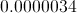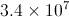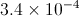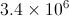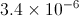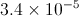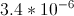## Example Questions

← Previous 1

### Example Question #1 : How To Use Scientific Notation

What is the sum of the solutions of the equation x2-2x-8=0?

-10

-2

-6

2

6

2

Explanation:

The equation factors to (x-4)(x+2)=0, indicating that the roots are x=-2,4. The sum of these two roots is 2.

### Example Question #2 : How To Use Scientific Notation

What is the number 350,000,000 in scientific notation?

3.5 x 105

3.5 x 106

3.5 x 108

3.5 x 107

3.5 x 109

3.5 x 108

Explanation:

350,000,000 = 3.5 x 108 (exponent is equal to the number of decimal points shifted)

### Example Question #3 : How To Use Scientific Notation

Given the equation 2xy + 4x² - y = 2x, if x = 2, what does y equal?

0

2

-4

4

-4

Explanation:

We plug in the value given for x and solve for y.

2xy + 4x² - y = 2x

2(2)y + 4(2)² - y = 2(2)

4y + 4(4) - y = 4

4y - y + 16 = 4

3y = -12

y = -4

### Example Question #4 : How To Use Scientific Notation

How do you express 657,800 in scientific notation?

6.578 × 103

65.78 × 106

65.78 × 103

65.78 × 104

6.578 × 104

65.78 × 104

Explanation:

657800 = 6.578 × 105

OR

657800 = 65.78 × 104

Exponent on 10 is the number of decimal points over the number has shifted.

### Example Question #1 : How To Use Scientific Notation

2 × 100 + 3 × 102 + 4 × 104 = ?

432

4,032

400,032

40,320

40,302

40,302

Explanation:

2 × 100 + 3 × 102 + 4 × 104 =

2 + 300 + 40,000 = 40,302

### Example Question #6 : How To Use Scientific Notation

Give this number in scientific notation: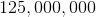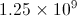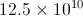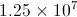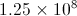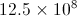Explanation:

There are six zeros and two more number places, for a total of eight, so 125,000,000 is equivalent to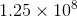.

### Example Question #1 : How To Use Scientific Notation

Simplify the following expression: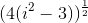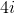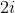Explanation: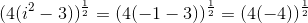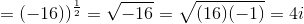### Example Question #231 : Exponents

What is the number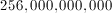in scientific notation?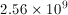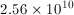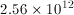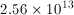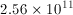Explanation: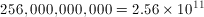The exponent is equal to the number of decimal points shifted.

### Example Question #240 : Exponents

Convert from scientific to decimal notation: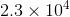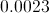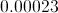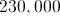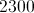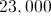Explanation:

In this case the exponent is positive hence you have to move the decimal point 4 places to the right from where it is in the problem giving us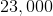### Example Question #8 : How To Use Scientific Notation

Convert from decimal to scientific notation: